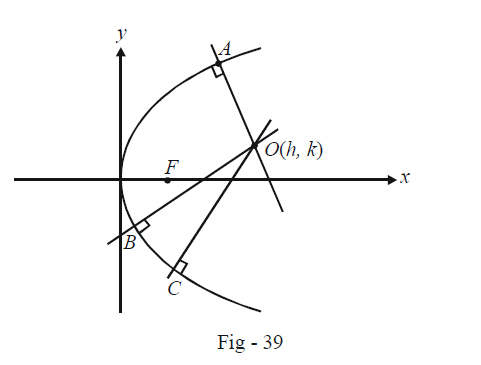# Parabolas Set 4

Go back to  'SOLVED EXAMPLES'

Example – 7

The normals at three points A, B and C on a parabola intersect at O. F is the focus of the parabola. Prove that

$FA\cdot FB \cdot FC = \frac{l}{4} \cdot F{O^2}$

where l is the length of the latus-rectum.

Solution: Assume the parabola to be $${y^2} = 4ax,$$ and the point O to be (h, k). F is the point (a, 0).Any normal to is $${y^2}= 4ax$$ is

$y = mx - 2am -a{m^3}$

and since this passes through O(h, k), we have

$k = mh - 2am -a{m^3}...\left( 1 \right)$

The feet of the three normal correspond to A, B and C. Thus, if $${m_1},\,{m_2}{\;\rm{and }}\;{\rm{ }}{m_3}$$ and are the roots of (1), the co-ordinates of A, B and C are

$(am_1^2,\, -2a{m_1}),\,(am_2^2,\, - 2a{m_2}){\;\rm{and}}\;{\rm{ }}(am_3^2,\, - 2a{m_3})$

We have now,

$FA = \sqrt {{{(a -am_1^2)}^2} + {{(2a{m_1})}^2}}$

$= a(1 + m_1^2)$

Similarly, $$FB = a(1 + m_2^2){\;\rm{ and}}\;{\rm{ }}FC = a(1 + m_3^2)$$.

Thus,

\begin{align}&FA \cdot FB \cdot FC= {a^3}(1 + m_1^2)(1 + m_2^2)(1 + m_3^2)\\\\&= {a^3}\left( {1 +m_1^2 + m_2^2 + m_3^2 + m_1^2m_2^2 + m_2^2m_3^2 + m_3^2m_1^2 +{{({m_1}{m_2}{m_3})}^2}} \right) \qquad \quad \dots \left( 2 \right)\end{align}

From(1), we know the values of

\left.\begin{align}{m_1} + {m_2} +{m_3} = 0\\{m_1}{m_2} +{m_2}{m_3} + {m_3}{m_1} = \left( {\frac{{2a - h}}{a}} \right)\\{m_1}{m_2}{m_3} = -\frac{k}{a}\end{align}\right\} \qquad \quad \dots\left( 3 \right)

Our task is to express the relation (2) in terms of the known quantities given by(3). This can be done as follows :

\begin{align}&\qquad \qquad \qquad \qquad m_1^2+ m_2^2 + m_3^3 = {({m_1} + {m_2} + {m_3})^2} - 2({m_1}{m_2} + {m_2}{m_3} +{m_3}{m_1})\\\\&m_1^2m_2^2 + m_2^2m_3^2 + m_3^2m_1^2 = {({m_1}{m_2} + {m_2}{m_3} +{m_3}{m_1})^2} - 2{m_1}{m_2}{m_3}({m_1} + {m_2} + {m_3})\end{align}

Substituting the appropriate values gives

\begin{align}FA\cdot FB \cdot FC &= {a^3}\left\{ {1 + {{\left( {\frac{{2a - h}}{a}} \right)}^2}- 2\left( {\frac{{2a - h}}{a}} \right) + \frac{{{k^2}}}{{{a^2}}}} \right\}\\\\ &=a\{ {(h - a)^2} + {k^2}\} \end{align}

which evidently equals $$a \cdot F{O^2}.$$ Since $$l = 4a,$$ we get the desired result:

$FA \cdot FB \cdot FC = \frac{l}{4} \cdot F{O^2}$

Example - 8

Normals are drawn form the point P with slopes $${m_1},{\rm{ }}{m_2},{m_3}$$ to the parabola $${y^2} = 4x.$$ If the locus of P with $${m_1}{m_2} = \alpha$$ is a part of the parabola itself,then find $$\alpha$$ .

Solution: If we let P be the point (h, k), we have

${m^3} + (2 - h)m + k = 0 \qquad \quad \dots \left( 1 \right)$

Thus,

\begin{align}&\qquad \;{m_1}{m_2}{m_3}= - k\\\\&\Rightarrow \quad {m_3} = - \frac{k}{\alpha }\left( {{\rm{since}}\;{m_1}{m_2} = \alpha } \right)\end{align}

Substituting this back into (1), we obtain

\begin{align}& \qquad \qquad - \frac{{{k^3}}}{{{\alpha ^3}}} - \frac{k}{\alpha }(2 - h) + k = 0\\\\&\Rightarrow \qquad \;\;{k^2} = {\alpha ^2}h - 2{\alpha ^2} + {\alpha ^3} \qquad \qquad \dots \left( 2\right)\end{align}

Also, since P lies on the parabola itself, we have

${k^2} =4h...\left( 3 \right)$

From(2) and (3), we have

$\begin{array}{l}{\alpha^2} = 4{\rm{ }}\;and\;{\rm{ }}{\alpha ^3} - 2{\alpha ^2} = 0\\\\\Rightarrow \alpha = 2\end{array}$

Learn from the best math teachers and top your exams

• Live one on one classroom and doubt clearing
• Practice worksheets in and after class for conceptual clarity
• Personalized curriculum to keep up with school